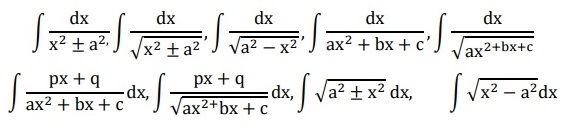Safalta expert Published by: Yashaswi More Updated Mon, 31 Jan 2022 08:16 PM IST

## Highlights

In the Term 2 CBSE Class 12 Maths board exam 2022, subjective questions will be asked. Students studying for the board exam in 2022 can use this syllabus to organize their studies. This is for students taking the Board Exam 2022 in Term 2.

The CBSE Class 12 Maths Syllabus 2022 for Term 2 may be seen below. This is for students taking the CBSE Class 12 Maths Board Exam 2022 in Term 2. In the Term 2 CBSE Class 12 Maths board exam 2022, subjective questions based on the Term 2 CBSE Class 12 Maths Syllabus will be asked. Students studying for the CBSE Class 12 Maths board exam in 2022 can use this syllabus to organize their studies. Join Safalta School Online and prepare for Board Exams under the guidance of our expert faculty. Our online school aims to help students prepare for Board Exams by ensuring that students have conceptual clarity in all the subjects and can score their maximum in the exams.

Also, Check-
To check the preparation tips for CBSE Class 12 English, click here.
To check the preparation tips for CBSE Class 12 Maths, click here.

### Syllabus for CBSE Class 12 Maths 2022 (Term 2): CBSE Class 12 Board Exams 2022

Check out the CBSE Class 12 Maths Syllabus for Term 2 in 2022 in the following table-
 No. Units Marks III Calculus 18 IV Vectors and Three-Dimensional Geometry 14 V Probability 8 Total 40 Internal Assessment 10 Total 50

Unit-III: Calculus

1.

### Free Demo ClassesSource: Safalta.com

Integrals

Integration is an inverse process of differentiation. Integration of a variety of functions by substitution, by partial fractions, and by parts, Evaluation of simple integrals of the following types and problems based on themFundamental Theorem of Calculus (without proof).Basic properties of definite integrals and evaluation of definite integrals.

2. Applications of the Integrals

Applications in finding the area under simple curves, especially lines, parabolas; area of circles /ellipses (in standard form only) (the region should be identifiable).

3. Differential Equations

Definition, order, and degree, general and particular solutions of a differential equation. Solution of differential equations by the method of separation of variables, solutions of homogeneous differential equations of the first order and first degree of the type: 𝑑𝑦/𝑑𝑥 = 𝑓(y/x). Solutions of linear differential equation of the type:

dy/dx + py = q, where p and q are functions of x or constant.

Also, Check-
CBSE Class 10 Model Papers for Term 1 Exam: Download In PDF
CBSE results delayed for TERM-1 EXAMS 2021-22

Unit-IV: Vectors and Three-Dimensional Geometry

1. Vectors

Vectors and scalars, magnitude and direction of a vector. Direction cosines and direction ratios of a vector. Types of vectors (equal, unit, zero, parallel and collinear vectors), position vector of a point, negative of a vector, components of a vector, the addition of vectors, multiplication of a vector by a scalar, position vector of a point dividing a line segment in a given ratio. Definition, Geometrical Interpretation, properties, and application of scalar (dot) product of vectors, vector (cross) product of vectors.

2. Three - dimensional Geometry

Direction cosines and direction ratios of a line joining two points. Cartesian equation and vector equation of a line, coplanar and skew lines, the shortest distance between two lines. Cartesian and vector equation of a plane. Distance of a point from a plane.

Unit-VI: Probability

1. Probability

Conditional probability, multiplication theorem on probability, independent events, total probability, Bayes’ theorem, Random variable, and its probability distribution.

 Internal Assessment 10 Marks Periodic Test 5 Marks Mathematics Activities: Activity file record +Term end assessment of one activity & Viva 5 Marks

Note: For activities, NCERT Lab Manual may be referred to.

Assessment of Activity Work:

In the first term, any 4 activities, and in the second term any 4 activities shall be performed by the student from the activities given in the NCERT Laboratory Manual for the respective class (XI or XII) which is published on the NCERT official website.

The weightage is as under:

The activities performed by the student in each term and record-keeping: 3 marks

Assessment of the activity performed during the term-end test and Viva-voce: 2 marks

For complete details of the CBSE Class 12 Maths Syllabus 2021-22 (Term 2) PDF, check this link.